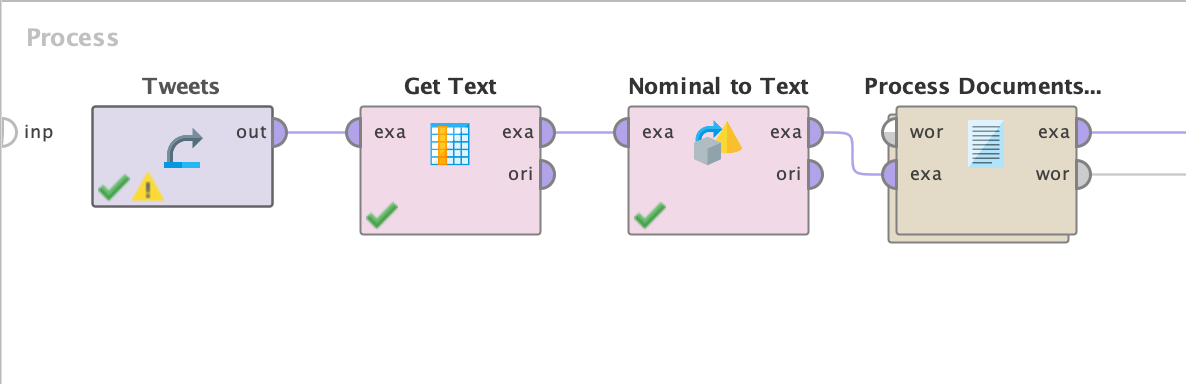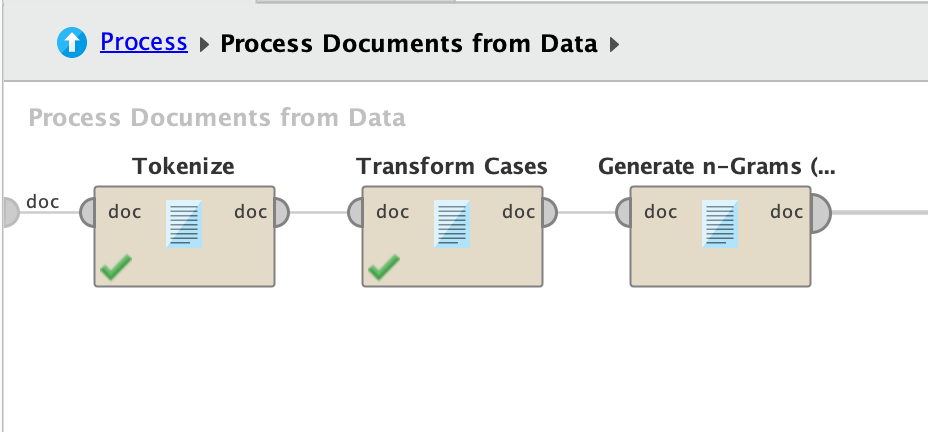# TWITTER: Splitting Text from Tweets

Member Posts: 1Newbie
edited June 2019 in Help
Hello, I'm Martin and I'm doing a homework regarding the Twitter analysis.
After getting the data, one cell containing text is providing me with many pieces of information which I would like to be separated or deleted.

For example:
RT @DefendingB: @Disinfo1982 @GeorgeFoulkes @CorinneJaneBrya I'll happily explain it to you. This edition was broadcast on 11th April 2014.…
or
 RT @GlosLiveOnline: Ann Widdecombe will headline Brexit Party rally at Gloucester Guildhall on night before European elections news from...I appositely selected these two (in bold the text I would desire), to show the difficulties I find in finding similarities among these to sentences for extracting them in a new cell. If you find a way to help me out, I'll be happy to spread out my gotten knowledge to othersTagged:

• Moderator, RapidMiner Certified Analyst, Member, University Professor Posts: 568Unicorn
Hello, @Martin_Novak_95

I don't know exactly what are you trying to accomplish, but for starters, I would check this:Inside the super operator Process Documents from Data, I have this:That is NLP 101. Then, you can Filter Stopwords (English), or play with POS Tagging, as pointers to do what you want. I'm sorry I can't be more helpful, but most of my NLP processing is done with Python (which, BTW, you can integrate here by using the Python Scripting Extension, if you want to be even more creative).
```<?xml version="1.0" encoding="UTF-8"?><process version="9.2.001">
<context>
<input/>
<output/>
<macros/>
</context>
<operator activated="true" class="process" compatibility="9.2.001" expanded="true" name="Process">
<parameter key="logverbosity" value="init"/>
<parameter key="random_seed" value="2001"/>
<parameter key="send_mail" value="never"/>
<parameter key="process_duration_for_mail" value="30"/>
<parameter key="encoding" value="SYSTEM"/>
<process expanded="true">
<operator activated="true" class="retrieve" compatibility="9.2.001" expanded="true" height="68" name="Tweets" width="90" x="45" y="34">
<parameter key="repository_entry" value="//Local Repository/data/tweets"/>
</operator>
<operator activated="true" class="select_attributes" compatibility="9.2.001" expanded="true" height="82" name="Get Text" width="90" x="179" y="34">
<parameter key="attribute_filter_type" value="single"/>
<parameter key="attribute" value="Text"/>
<parameter key="attributes" value=""/>
<parameter key="use_except_expression" value="false"/>
<parameter key="value_type" value="attribute_value"/>
<parameter key="use_value_type_exception" value="false"/>
<parameter key="except_value_type" value="time"/>
<parameter key="block_type" value="attribute_block"/>
<parameter key="use_block_type_exception" value="false"/>
<parameter key="except_block_type" value="value_matrix_row_start"/>
<parameter key="invert_selection" value="false"/>
<parameter key="include_special_attributes" value="false"/>
</operator>
<operator activated="true" class="nominal_to_text" compatibility="9.2.001" expanded="true" height="82" name="Nominal to Text" width="90" x="313" y="34">
<parameter key="attribute_filter_type" value="all"/>
<parameter key="attribute" value=""/>
<parameter key="attributes" value=""/>
<parameter key="use_except_expression" value="false"/>
<parameter key="value_type" value="nominal"/>
<parameter key="use_value_type_exception" value="false"/>
<parameter key="except_value_type" value="file_path"/>
<parameter key="block_type" value="single_value"/>
<parameter key="use_block_type_exception" value="false"/>
<parameter key="except_block_type" value="single_value"/>
<parameter key="invert_selection" value="false"/>
<parameter key="include_special_attributes" value="false"/>
</operator>
<operator activated="true" class="text:process_document_from_data" compatibility="8.1.000" expanded="true" height="82" name="Process Documents from Data" width="90" x="447" y="34">
<parameter key="create_word_vector" value="true"/>
<parameter key="vector_creation" value="TF-IDF"/>
<parameter key="keep_text" value="true"/>
<parameter key="prune_method" value="none"/>
<parameter key="prune_below_percent" value="3.0"/>
<parameter key="prune_above_percent" value="30.0"/>
<parameter key="prune_below_rank" value="0.05"/>
<parameter key="prune_above_rank" value="0.95"/>
<parameter key="datamanagement" value="double_sparse_array"/>
<parameter key="data_management" value="auto"/>
<parameter key="select_attributes_and_weights" value="false"/>
<list key="specify_weights"/>
<process expanded="true">
<operator activated="true" class="text:tokenize" compatibility="8.1.000" expanded="true" height="68" name="Tokenize" width="90" x="45" y="34">
<parameter key="mode" value="non letters"/>
<parameter key="characters" value=".:"/>
<parameter key="language" value="English"/>
<parameter key="max_token_length" value="3"/>
</operator>
<operator activated="true" class="text:transform_cases" compatibility="8.1.000" expanded="true" height="68" name="Transform Cases" width="90" x="179" y="34">
<parameter key="transform_to" value="lower case"/>
</operator>
<operator activated="true" class="text:generate_n_grams_terms" compatibility="8.1.000" expanded="true" height="68" name="Generate n-Grams (Terms)" width="90" x="313" y="34">
<parameter key="max_length" value="2"/>
</operator>
<connect from_port="document" to_op="Tokenize" to_port="document"/>
<connect from_op="Tokenize" from_port="document" to_op="Transform Cases" to_port="document"/>
<connect from_op="Transform Cases" from_port="document" to_op="Generate n-Grams (Terms)" to_port="document"/>
<connect from_op="Generate n-Grams (Terms)" from_port="document" to_port="document 1"/>
<portSpacing port="source_document" spacing="0"/>
<portSpacing port="sink_document 1" spacing="0"/>
<portSpacing port="sink_document 2" spacing="0"/>
</process>
</operator>
<connect from_op="Tweets" from_port="output" to_op="Get Text" to_port="example set input"/>
<connect from_op="Get Text" from_port="example set output" to_op="Nominal to Text" to_port="example set input"/>
<connect from_op="Nominal to Text" from_port="example set output" to_op="Process Documents from Data" to_port="example set"/>
<connect from_op="Process Documents from Data" from_port="example set" to_port="result 1"/>
<connect from_op="Process Documents from Data" from_port="word list" to_port="result 2"/>
<portSpacing port="source_input 1" spacing="0"/>
<portSpacing port="sink_result 1" spacing="0"/>
<portSpacing port="sink_result 2" spacing="0"/>
<portSpacing port="sink_result 3" spacing="0"/>
</process>
</operator>
</process>
```

Hope this points you on the right direction.

All the best,

Rodrigo.